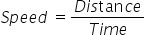Mathematics
Easy

Question

# A minibus drives with a constant speed of 45 miles in 3 hours. If it travels at the same rate, distance covered by the bus in 4 hours would be _________________.

## 25315160Hint:

## The correct answer is: 60

### The given values of distance and time are as follows:Distance = 45 milesTime = 3 hoursWe have to find the speed as it is given that speed is constant.We know the formula for speed.Now, as the rate or speed is constant we can use this value to find the value of distance travelled for any other time.We have to find the distance travelled in 4 hours.Let’s see the formulaRearranging the formula to find distance. We will multiply both sides by time.Distance = Speed × Time= 15 miles/ hour × 4 hours= 60 milesTherefore, the distance travelled is 60 miles.So the option which is ‘60’, is the right option.

For such questions, we should be careful about the units. Same quantities must have same units.

### Related Questions to study#### With Turito Foundation.#### Get an Expert Advice From Turito.